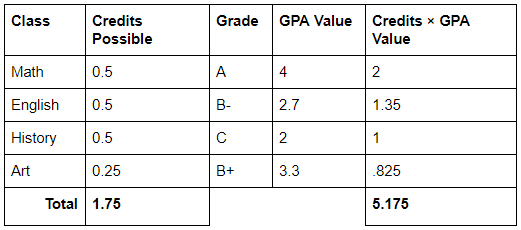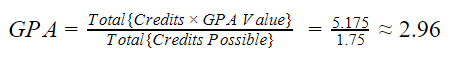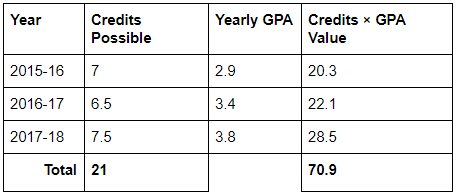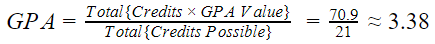# How are GPAs calculated?

Grade point averages (GPAs) are used as a quick way to assess and rank student performance across all classes. This article discusses the typical 4.0 or 5.0 system used by most high schools. For details on percentage-based GPAs, see How Are Percentage-Based GPAs Calculated?

In a typical 4.0 or 5.0 GPA system, each letter grade is worth a specific number of points (the GPA Value, also called grade points). The GPA for each grading period is determined by the letter grades on the report card for that grading period. Read about how to control which courses count toward GPA.

This system allows schools to control how much weight each class has (by editing the number of credits on the course) and to specify how many grade points each letter grade represents (by editing the grading scale used on the course).

Below is a table presenting information about a sample student’s report card grades during one grading period and an formula demonstrating how the GPA is calculated.## Regular, Summary, and Cumulative GPA

Regular grading periods are usually quarters, trimesters, or exam grading periods. Each regular grading period has its own gradebook and report card scores and is independent of other grading periods. GPA for regular grading periods is calculated from report card grades as explained in the example above.

Summary grading periods include semesters and final grading periods. They have report cards grades but no gradebook grades associated with them. Consequently, a summary GPA is calculated from report card grades exactly as it is calculated for a regular grading period. It is a common error to assume that the GPA for Sem #1, for instance, is the average of the previous Q1 and Q2 GPAs.

Yearly GPA indicates the GPA value for the whole academic year. It is calculated from all the highest-level report card grades depending on the school’s grading period system:

• School has a final grading period
• The Yearly GPA will be identical to the Final GPA; both are calculated from the report card grades in the final grading period.
• School has semesters but no final
• The Yearly GPA will be calculated by applying the formula to all Sem #1 and Sem #2 report card grades.
• School only has regular grading periods (no semesters nor final)
• The Yearly GPA will be calculated by applying the formula to all report card grades throughout the year.

Cumulative GPA represents the GPA value across multiple academic years. It is not a straight average of yearly GPA across those academic years. Instead, cumulative GPA is calculated by looking at each year’s Credits Possible, Yearly GPA, and Credits * GPA Value. We take the sum of all (Credits Possible * Yearly GPA) and divide that by the number of Credits Possible. The cumulative GPA will not be changed until summary grades are entered (if summary grading period grades exist at your school). Here is an example of the cumulative GPA of a student who has completed three years.## Weighted GPA

If your school calculates weighted and unweighted GPAs separately, each letter grade from the grading scale will have a separate value for Weighted GPA and Unweighted GPA. Weighted GPAs are typically used to give students more grade points for taking difficult classes. For example, a student who takes AP Calculus may ordinarily receive 4 grade points for getting an A. On a weighted scale, the student might receive 5 grade points. The calculation formula does not change, but the student receives a higher GPA for taking a more difficult class.

Both weighted and unweighted GPA Values are entered on the grading scale. When calculating GPA, you can switch between Weighted and Unweighted GPA modes. The unweighted GPA mode uses unweighted GPA values for all courses. The weighted GPA mode uses weighted GPA value for weighted courses and unweighted GPA values for unweighted courses. Both GPAs can be shown or hidden from report cards, and either can be used when running reports.